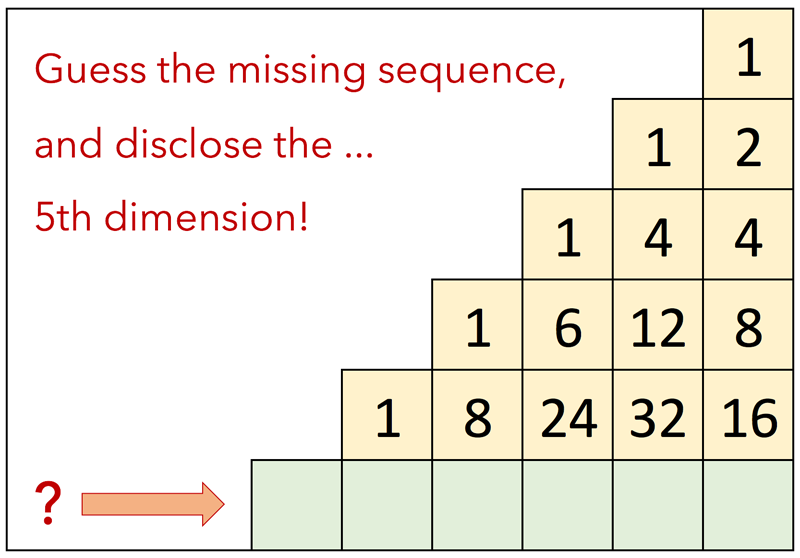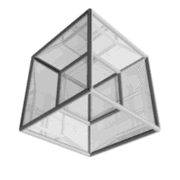# Gaetano Causio## Can you find the missing number sequence?

Don't be misled by the simple linear thinking of other
similar guessing games...

you need a multidimensional approach to solve this one!

## Solution: The 5th Dimension

Before jumping to the mere formula, let's have a look at what above matrix represents in a multidimensional world.

The first number on top of our matrix (1) represents a dot, a single point with no sizes in a world without dimensions, the whole universe in a dot, a singularity, for some the beginning of our universe itself, but this is a different story. Clone, duplicate our dot and you'll get two dots, connect the dots together and here you have a line lying on a one dimensional space, and that's the second sequence on our matrix (1 - 2), do the same on the third sequence: clone the line, connect the edges and... got it?

Yeah, the third sequence represents a square in a two dimensional space: 4 dots or vertices connected by 4 lines shaping 1 square (1 - 4 - 4). Logically the fourth sequence (1 - 6 - 12 - 8) represents a cube composed by 8 vertices, 12 lines and 6 faces in a three dimensional space.And now it becomes interesting: using the same analogy, the next sequence would represents a cube in a four dimensional space (a tesseract) and therefore the missing sequence represents a hypercube in the fifth dimension! Left, a 3D image of a Tesseract shadow while rotating in its own 4th dimension. Yes, we can only visualize its shadow! Fascinating, isnt it?

The Formula

Each cell in our matrix represents the property (in terms of edges, lines, faces, etc.) of a shape in a given spatial n dimension. And we've seen that a shape in a nth dimensional space is created by cloning/duplicating the corresponding shape in the previous (n - 1) dimension and by connecting all vertices of the two shapes. Take the number 6 in the the 3rd dimension, it represents the six faces of a cube and can be constructed by cloning the corresponding square in the 2nd dimension (1 + 1) and by connecting all edges with 4 lines. At the same way, the 32 lines of our tesseract in the 4th dimension are given by cloning the 12 lines from the shape of the previous dimension (12 + 12) and by adding the corresponding connections (8). So 6 = 2*1 + 4 and 32 = 2*12 + 8.

S(p,n) = 2 * S(p, n-1) + S(p-1 ,n-1)

S = Shape in a cell
p = Property coordinate (column index in our matrix - from right to left)
p = Dimension coordinate (row index in our matrix - from top to bottom)

Or simply: each number in a cell (ie 32) is given by doubling the number in the cell of the previous row (2 * 12) and adding the one from this latter right adjacent cell (8). The missing sequence is then: 1 10 40 80 80 32